Courses

# Test: Rotational Motion (Competition Level 1) - 1

## 30 Questions MCQ Test Physics Class 11 | Test: Rotational Motion (Competition Level 1) - 1

Description
This mock test of Test: Rotational Motion (Competition Level 1) - 1 for Class 11 helps you for every Class 11 entrance exam. This contains 30 Multiple Choice Questions for Class 11 Test: Rotational Motion (Competition Level 1) - 1 (mcq) to study with solutions a complete question bank. The solved questions answers in this Test: Rotational Motion (Competition Level 1) - 1 quiz give you a good mix of easy questions and tough questions. Class 11 students definitely take this Test: Rotational Motion (Competition Level 1) - 1 exercise for a better result in the exam. You can find other Test: Rotational Motion (Competition Level 1) - 1 extra questions, long questions & short questions for Class 11 on EduRev as well by searching above.
QUESTION: 1

### The moment of inertia of a body depends upon -

Solution:

I = mr2, therefore it depends on body mass and its mass distribution.

QUESTION: 2

### Two spheres of same mass and radius are in contact with each other. If the moment of inertia of a sphere about its diameter is I, then the moment of inertia of both the spheres about the tangent at their common point would be -

Solution:

The moment of inertia of a sphere about its diameter is given as,
I=2​/5 MR2
The moment of inertia of the sphere about the tangent is given as,
I′=2/5​MR2+MR2
I′=7/5​MR2
The total moment of inertia of both spheres about the common tangent is given as,
​It=2I′
It​=2×7/5​MR2
It​=7I

QUESTION: 3

### A disc of metal is melted to recast in the form of a solid sphere. The moment of inertias about a vertical axis passing through the centre would -

Solution:

Moment of inertia will decrease, because
Id=1/2mr2 and Is=2/5mr2,
the radius of sphere formed on recasting the disc will also decrease.

QUESTION: 4

The M.I. of a disc about its diameter is 2 units. Its M.I. about axis through a point on its rim and in the plane of the disc is

Solution:

We know that for a disc of mass m and radius r
MI of a disc about its diameter = mr2/4 = 2
And also MI about a point on its rim = mr2/4 + mr2
= 5mr2/4
= 5 x 2 = 10

QUESTION: 5

A solid sphere and a hollow sphere of the same mass have the same moments of inertia about their respective diameters, the ratio of their radii is

Solution:

We know moment of inertia of solid sphere Is​=2​/5ms​Rs2​ and
moment of inertia of hollow sphere IH​=2/3​mH​RH2 ​As per question Is​=IH​
Now,
2/5​ms​Rs2​=2/3​mH​RH2​
as the masses are equal the ratio of their radii will be
​Rs2 /RH2 ​​=2/3​/​2/5​=√5/3​​=(5)1/2: (3)1/2

QUESTION: 6

A stone of mass 4kg is whirled in a horizontal circle of radius 1m and makes 2 rev/sec. The moment of inertia of the stone about the axis of rotation is

Solution:

Moment of Inertia = MR²
= 4 × 1
= 4 kg-m²

QUESTION: 7

Three rings, each of mass P and radius Q are arranged as shown in the figure. The moment of inertia of the arrangement about YY' axis will be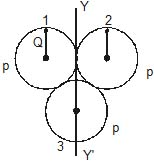Solution:

For ring 1
(MOI)1 = MOI about diameter + PQ2
MOI about diameter = ½ PQ2
(MOI)1 = 3/2 PQ2
Similarly, (MOI)2 = 3/2 PQ2
(MOI)3 = ½ PQ2
Total MOI = 7/2 PQ2

QUESTION: 8

A circular disc A of radius r is made from an iron plate of thickness t and another circular disc B of radius 4r is made from an iron plate of thickness t/4. The relation between the moments of inertia IA and IB is

Solution: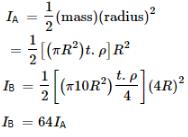QUESTION: 9

The moment of inertia of a uniform semicircular wire of mass M and radius r about a line perpendicular to the plane of the wire through the centre is

Solution:

The formula for the MOI of a uniform semicircular ring about a perpendicular line is ½ Mr2

QUESTION: 10

Let IA and IB be moments of inertia of a body about two axes A and B respectively. The axis A passes through the centre of mass of the body but B does not.

Solution:

If the axes are parallel, we use the formula:
Io = Icm + md2
For the first body the distance between the axis and the axis passing through C.O.M is 0
Therefore, Io = Icm + m(0)2 = lcm since it is mentioned in question
Whereas for the second body it is not passing through the C.O.M therefore, there is some distance between the axes, say 'd'
So, Io = Icm + md2

QUESTION: 11

For the same total mass which of the following will have the largest moment of inertia about an axis passing through its centre of mass and perpendicular to the plane of the body

Solution:

Moment of inertia depends on distribution of mass around axis. The more the mass near the axis lesser is the moment of inertia. Four rods forming a square have more moment of inertia because of less mass near axis.

QUESTION: 12

Moment of inertia of a thin semicircular disc (mass = M & radius = R) about an axis through point O and perpendicular to plane of disc, is given by :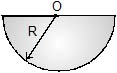Solution:

Mass of semicircular disc = M
Suppose there is a circular disc of mass 2M, then
Moment of intertia of circular disc = ½ (2M)R2
Moment of intertia of circular disc = ½ (2M)R2 = MR2
=> So, Moment of intertia of semi-circular disc = ½ MR2

QUESTION: 13

A rigid body can be hinged about any point on the x-axis. When it is hinged such that the hinge is at x, the moment of inertia is given by

I = 2x2 - 12x + 27 The x-coordinate of centre of mass is

Solution:

Moment of inertia is minimum at centre of mass of the body.
I = minimum for centre of mass
Therefore x co-ordinate of centre of mass is 3 unit.

QUESTION: 14

Consider the following statements

Assertion (A) : The moment of inertia of a rigid body reduces to its minimum value as compared to any other parallel axis when the axis of rotation passes through its centre of mass.

Reason (R) : The weight of a rigid body always acts through its centre of mass in uniform gravitational field. Of these statements :

Solution:

Moment of inertia about its centroidal axis has a minimum value as the centroidal axis has mass evenly distributed around it thereby providing minimum resistance to rotation as compared to any other axis. Also the statement-2 is correct but is not the correct explanation for statement-1.

QUESTION: 15

A body is rotating uniformly about a vertical axis fixed in an inertial frame. The resultant force on a particle of the body not on the axis is

Solution:

Since the axis is vertical, the force will be horizontal and since it is centripetal force it will intersect the axis.

QUESTION: 16

One end of a uniform rod of mass m and length I is clamped. The rod lies on a smooth horizontal surface and rotates on it about the clamped end at a uniform angular velocity w. The force exerted by the clamp on the rod has a horizontal component

Solution:

Consider a rod of length l and mass m rotating with an angular velocity of w
dF = dm l w2
dF = (m/l) dμ l w2
Integrating
F = ½mlw2

QUESTION: 17

A rod of length 'L' is hinged from one end. It is brought to a horizontal position and released. The angular velocity of the rod when it is in vertical position is

Solution: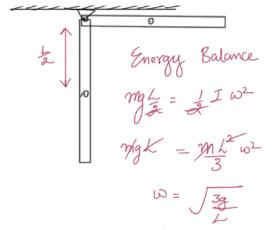QUESTION: 18

A disc of radius 2m and mass 200kg is acted upon by a torque 100N-m. Its angular acceleration would be

Solution:

F = ma
a = Wr
So, F = mwr
a = wr = acceleration
m = 200kg
F = 100 n-m, r = 2m, w = to be calculated
100 = 200 x w2

*Multiple options can be correct
QUESTION: 19

On applying a constant torque on a body

Solution:

If a constant torque is applied it is possible that a positive angular acceleration gets generated which can generate a positive acceleration and hence increasing both velocity and angular velocity.

QUESTION: 20

A wheel starting with angular velocity of 10 radian/sec acquires angular velocity of 100 radian/sec in 15 seconds. If moment of inertia is 10kg-m2, then applied torque (in newton-metre) is

Solution:

I(ωf - ωi)/t = τ
Hence, τ = 60

QUESTION: 21

An automobile engine develops 100H.P. when rotating at a speed of 1800 rad/min. The torque it delivers is

Solution:
QUESTION: 22

The moment of inertia and rotational kinetic energy of a fly wheel are 20kg-mand 1000 joule respectively. Its angular frequency per minute would be -

Solution:

Rotational K.E. = ½ × moment of inertia × square of angular velocity = ½Iw2

QUESTION: 23

Calculate the M.I. of a thin uniform ring about an axis tangent to the ring and in a plane of the ring, if its M.I. about an axis passing through the centre and perpendicular to plane is 4 kg m2.

Solution:

At tangent I =MR2/2 + MR2 --- (1)
MR2/2 = 4, MR2 = 8 then substitute in (1) you will get I = 12, this is for tangent perpendicular to plane then divide by 2 you will get tangent along the plane

QUESTION: 24

A torque of 2 newton-m produces an angular acceleration of 2 rad/sec2 a body. If its radius of gyration is 2m, its mass will be:

Solution: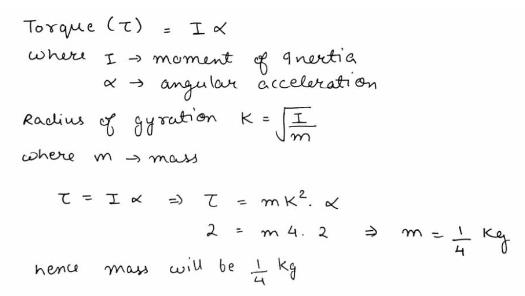QUESTION: 25

A particle is at a distance r from the axis of rotation. A given torque t produces some angular acceleration in it. If the mass of the particle is doubled and its distance from the axis is halved, the value of torque to produce the same angular acceleration is

Solution:

We know that from some torque t, angular acceleration a produced can be find by,
t = I a, where I is moment of inertia = mr2
Thus we get a = t / mr2
Now we have 2m and r/2
Thus a =  t / mr2 = T / 2m(r/2)2
Thus we get T = t/2

QUESTION: 26

A weightless rod is acted on by upward parallel forces of 2N and 4N ends A and B respectively. The total length of the rod AB = 3m. To keep the rod in equilibrium a force of 6N should act in the following manner :

Solution: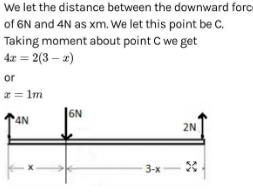QUESTION: 27

A right triangular plate ABC of mass m is free to rotate in the vertical plane about a fixed horizontal axis through A. It is supported by a string such that the side AB is horizontal. The reaction at the support A is :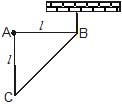Solution:

The distance of Centre Of Mass of the given right angled triangle is 2L/3​ along BA and L/3​ along AC from the point B.
Force of magnitude mg is acting downwards at its COM.
Moment balance around B gives:
mg(2L/3​)−FA​(L)=0
(Moment=  × =rFsin(θ)=F(rsin(θ))=Fr⊥​)
∴FA​=2​mg/3

QUESTION: 28

In an experiment with a beam balance on unknown mass m is balanced by two known mass m is balanced by two known masses of 16 kg and 4 kg as shown in figure.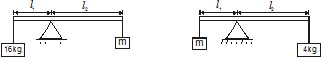The value of the unknown mass m is

Solution: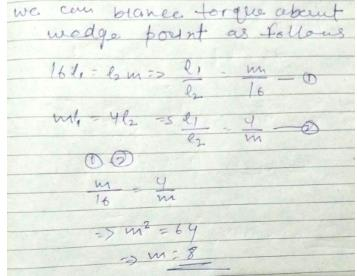QUESTION: 29

A homogeneous cubical brick lies motionless on a rough inclined surface. The half of the brick which applies greater pressure on the plane is :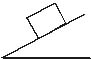Solution:

As weight of the body (mg) acts along the left side i.e. mg sinx acts along flow of object.
mgcosx acts perpendicular to flow of the object.
The pressure of right half comes on left half hence left half has maximum pressure.

QUESTION: 30

Consider the following statements

Assertion (A) : A cyclist always bends inwards while negotiating a curve

Reason (R) : By bending he lowers his centre of gravity Of these statements,

Solution:

B is correct. The reason is a part of the question.